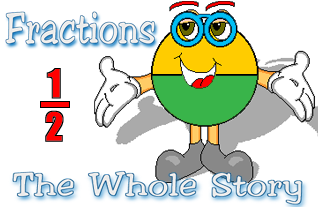An Introduction to Fractions for your iPhone...

In the era of computers and calculators, why is it important for students to learn fractions? A student must understand fractions in order to learn algebra and geometry. In addition to recognizing the parts of a fraction, young learners need to understand the meaning of each part and its relationship to the whole. Because many young learners have difficulty developing the concept of fractions, using pictures of shapes and groups of objects to represent fractions enables young learners to understand their meaning. Then, students can make the transition from the pictorial representation of fractions to abstract mathematical notation. This understanding allows them to move on to more advanced mathematics.

First, it is important for the child to understand that traditional representations of fractions have three parts: the numerator, the fraction bar and the denominator. Proper fractions are used to represent parts of a whole. The denominator tells into how many equal parts the whole is divided. The numerator tells how many of those parts are being considered. The fraction bar is a symbol for division.

Usually basic fractions concepts are represented in one of two ways; either by a shape divided into equal parts with some of the parts shaded, or by presenting a group of objects with some of the objects different in some way. Children should recognize both representations for fractions. For example, dividing a circle into four equal parts is one way to show the fraction one-fourth. Another way is to have a group of four objects with one or more of the objects different in some way.

Strong multiplication skills are a prerequisite to understanding and using fractions. In order to use Least Common Multiple (LCM) to compare and add fractions students must know the multiples of two numbers. LCM is used for finding equivalent fractions with common denominators.

How can you help a young learner understand fractions? Talk about the meaning of fractions by pointing out examples in your environment. How many cars in a row of the parking lot? What fraction are white cars? Point out how fractions are used when following a recipe or measuring wood for a building project. Practice multiplication by counting aloud together by two’s, three’s, and four’s, etc. Compare two sets of multiples (for example, 4,8,12,16, 20, 24... and 5, 10, 15, 20, 25...) and let your child discover the first number that appears in both sets. This number is the LCM. You’ll be amazed at how much a student will learn just by talking about fractions in everyday life.

How quickly students learn to work with fractions depends on many factors. But once they have mastered fractions, they will be ready to take on the challenges of higher math, especially algebra and geometry.

Fractions: The Whole Story is a fun, convenient way to introduce and reinforce basic fraction concepts. For all the times you and your student have a minute or two, this iPhone application is the perfect opportunity to practice multiplication skills. It will entertain both of you while you’re waiting in line at the grocery store, sitting in the waiting room at the dentist, or traveling by bus or train. The possibilities are endless!

 Features: Shapes and sets of object appear on the screen and then fade to screens labeled with fractions. Each fraction is pronounced. A quiz provides practice in recognizing fraction concepts. Lessons focused on basic concepts and equivalency are presented. Sequential/Random option determines the selection of Flash Cards. On/Off option controls sound effects. \$0.99
Activities

Fraction Flash Cards

Fraction Flash Cards help to develop an understanding of basic fraction concepts. Illustrated representations of fractions appear on the screen and dissolve into a card labeled with the related fraction concept. The flash card presentation focuses on understanding the meaning of a fraction and equivalency. At the basic level the terms'numerator' and 'denominator' are introduced. When using the flash cards, fractions are pronounced aloud as each card appears.

The child controls the pace of the learning experience by either swiping or tapping the screen to move to the next flash card. Shaking the iPhone causes another flash card to be selected randomly.

The Preferences screen provides control over a variety of options. Sound effects can be turned on and off. Whether the factors and products are spoken can also be controlled. To increase the difficulty and move from a representational learning stage to an abstract learning stage, numerals can be shown instead of counters.

The "Basic" vs. "Advanced" preference setting controls this option.

The Quiz

The Quiz is an interactive game where the child touches a button that is labeled with the correct answer to a question about fractions.

When the correct button is touched, a star appears on the screen. If an incorrect answer is selected, a star is removed from the screen.

The object of this activity is to get 10 stars.

Shaking the phone causes a different problem to be randomly selected.

When introducing the fraction concept by playing this game, encourage the child to recall the information presented in the lesson and also in the flash card activities.Lessons

To use the Fractions Lessons option, tap the right arrow or swipe to advance the lesson. Tap the left arrow or swipe to move to the previous page.

As in the Flash Card activity, the quiz and the lessons have two levels, basic and advanced. The "Basic" vs. "Advanced" preference settings control this option.

Tap home to exit the flash cards, quizzes or lessons.

Fractions: The Whole Story is now available at the Apple iTunes Store.